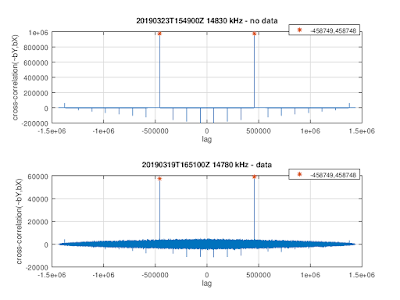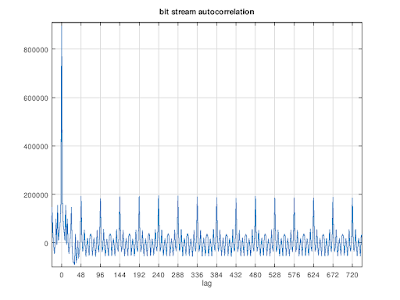## Sunday, March 24, 2019

### Interesting MSK-modulated signals on HF (2)

This is an update of the last blog post.

Yesterday, another signal was picked up for which both the "X" and the "Y" bit streams were generated by the same LFSR with polynomial [3,1,0] × [17,16,15,14,13,12,10,8,5,4,0] and period N= (23-1)×(217-1)=917,497. The cross-correlation between "X" and "Y" show that the "X" bit stream is offset w.r.t. "Y" bit stream by M=(N-1)/2=458,748 bits."X" and "Y" bit stream cross-correlations

The data in the "X" channel can descrambled as follows
b(i) = XOR(~Y(M+i), X(i)),
and the auto-correlation of the descrambled bits b(i) has peaks at multiples of 48 bits:Autocorrelation of the descrambled bit stream
In terms of 240 bit frames the descrambled bits look like this:Descrambled bit stream in terms of 240-bit frames

and in terms of 48-bit frames:Descrambled bit stream in terms of 48-bit frames
Here one can see that the descrambled bits come in pairs
b(2i) = b(2i+1)
so there are 24 pairs of bits per 48-bit frame. These 24 bits are not independent: they are determined by 6 bits only:
1  ~3    4  6 13  ~20
2   9  ~15
5  18 -~21
7   8   11 16 19 -~23
10  17
12  14 -~22
where ~P denotes logical NOT of bit number P and -P denotes a bit at position P from the previous frame.

These 6 bits are likely not independent. Interpreting them as a 6-bit binary number, the histogram of these numbers shows that they are not equally probable:Histogram of 6-bit frame interpreted as 6-bit binary numbers